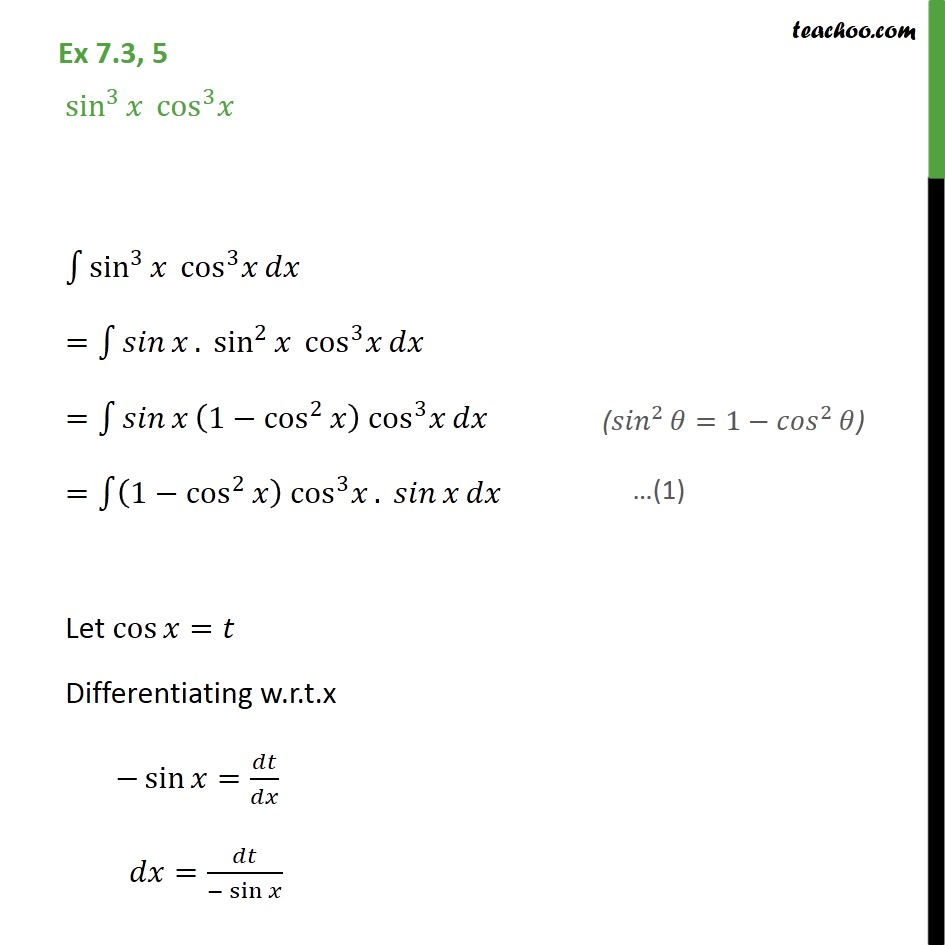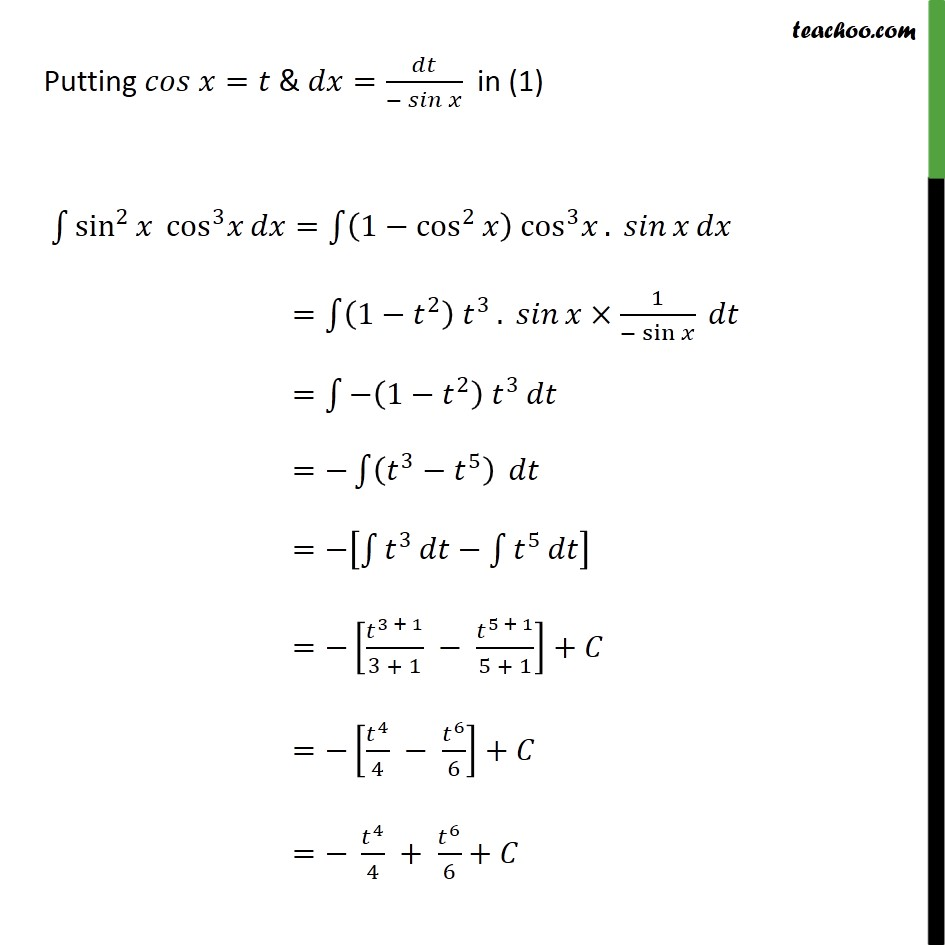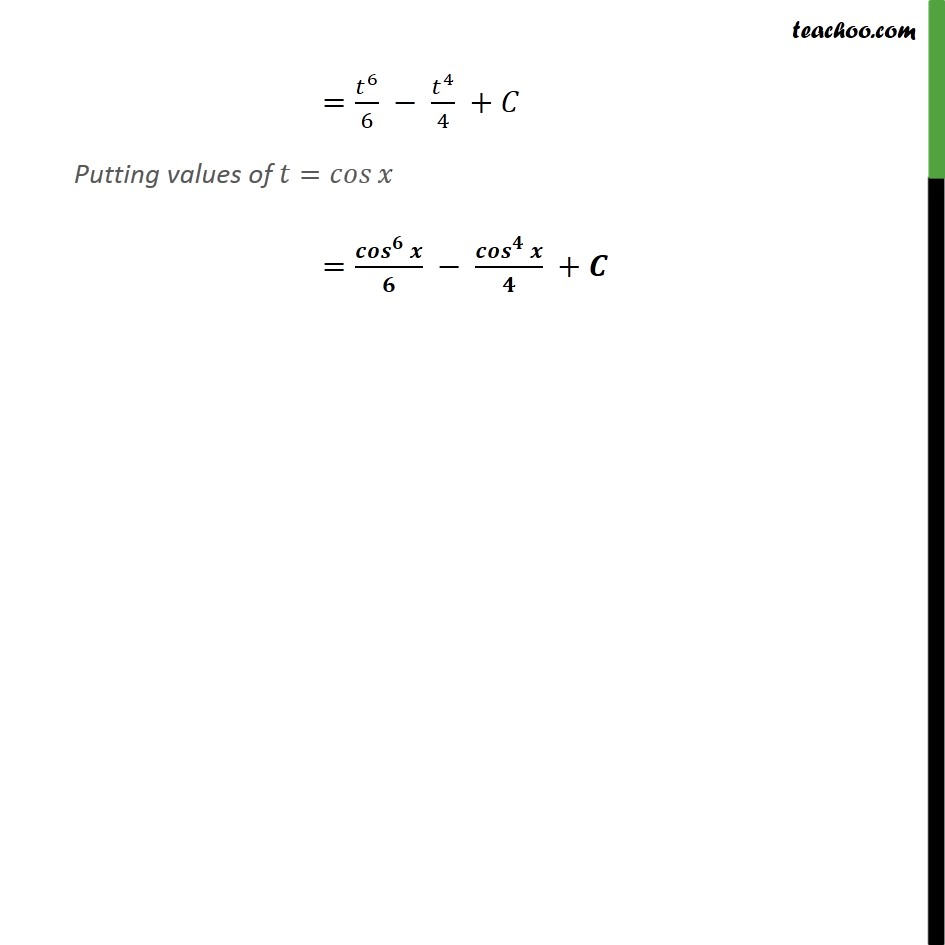1. Chapter 7 Class 12 Integrals
2. Serial order wise
3. Ex 7.3

Transcript

Ex 7.3, 5 sin^3 cos^3 1 sin^3 cos^3 = 1 . sin^2 cos^3 = 1 (1 cos^2 ) cos^3 = 1 (1 cos^2 ) cos^3 . Let cos = Differentiating w.r.t.x sin = / = /( sin ) Putting = & = /( ) in (1) 1 sin^2 cos^3 = 1 (1 cos^2 ) cos^3 . " " = 1 (1 ^2 ) ^3 . 1/( sin ) " " = 1 (1 ^2 ) ^3 " " = 1 ( ^3 ^5 ) " " = [ 1 ^3 1 ^5 ] = [ ^(3 + 1)/(3 + 1) ^(5 + 1)/(5 + 1)]+ = [ ^4/4 ^6/6]+ = ^4/4 + ^6/6+ = ^6/6 ^4/4 + Putting values of = =( ^ )/ ( ^ )/ +

Ex 7.3

Chapter 7 Class 12 Integrals
Serial order wise